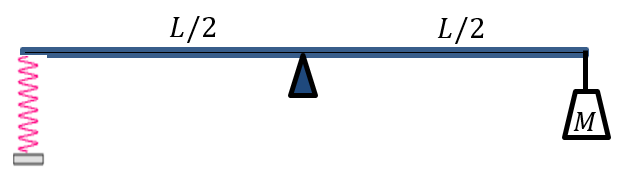# Rod in Static Equilibrium#

The figure shows a mass $$M$$ attached to the right end of a rod of length $$L$$ and of mass $$m$$. There is a support located at the middle of the rod. The left end of the rod is connected to a spring. The system is in static equilibrium.## Part 1#

Determine the magnitude of the vertical force exerted on the rod by the spring ($$F_S$$).

Use the following table as a reference for each variable. Note that it may not be necessary to use every variable.

For

Use

$$M$$

M

$$L$$

L

$$m$$

m

$$g$$

g

Please enter in a symbolic value in $$N$$.

## Part 2#

Determine the direction, $$\theta$$, of the vertical force exerted on the rod by the spring ($$F_S$$). Assume that the left end of the rod is at the origin of a Cartesian plane ($$\theta > 0$$ with zero being to the right).

Please enter in a numeric value in degrees.

## Part 3#

Find the magnitude of the normal force exerted on the rod by the support ($$n$$).

Use the following table as a reference for each variable. Note that it may not be necessary to use every variable.

For

Use

$$M$$

M

$$L$$

L

$$m$$

m

$$g$$

g

Please enter in a symbolic value in $$N$$.

## Part 4#

On the figure, indicate which pivot point have you chosen to answer Part 1. In addition, show the direction of $$F_S$$ determined in Part 2.

Your file must be a pdf.